# Work-Energy Derivation question: F(x(t)) = F(t) ?

Does this derivation: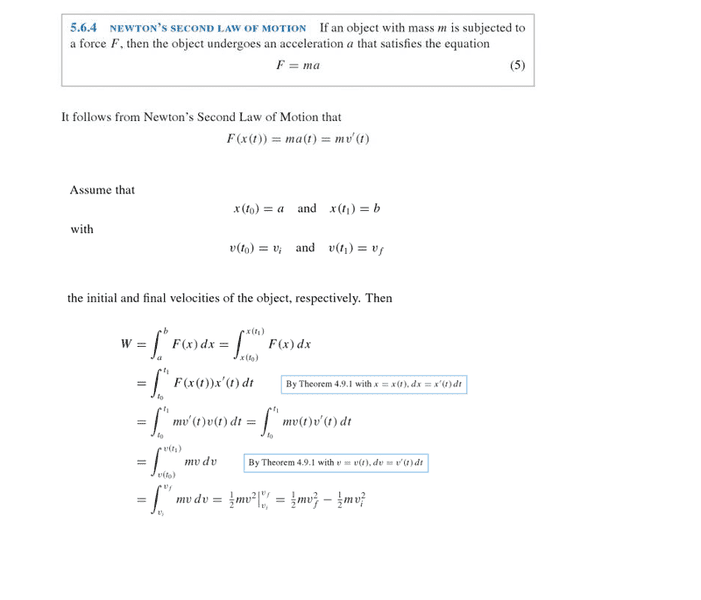...imply: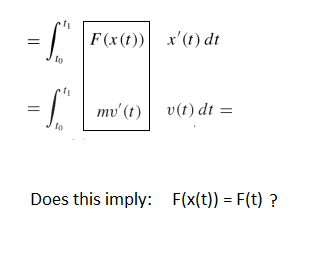My best guess is that x(t) ≠ t
So I would also guess that F(x(t)) ≠ F(t)

But then how can this derivation be explained?

How can F(x(t)) = m(a(t))? What does that actually mean?
How come it's not: F(x(t)) = m(a(x(t))) ? Why/How does the x just cancel out?

Thank you

Would this question be better suited for the math section? If so, please feel free to transfer it. It seems to be bordering on a math related question within a physics derivation and I don't think I'll fully understand the derivation without understanding how the math is being work at this part.

Again, thanks.

256bits
Gold Member
How can F(x(t)) = m(a(t))? What does that actually mean?

x(t) implies x is a function of t, ie for a particular t there is a particular x.
F(x) implies that F is a function of x
F(x(t)) implies ... You should be able to figure that out.
Same for a(t).

My best guess is that x(t) ≠ t
So I would also guess that F(x(t)) ≠ F(t)
Hmmmm

•Ocata
x(t) implies x is a function of t, ie for a particular t there is a particular x.
F(x) implies that F is a function of x
F(x(t)) implies ... You should be able to figure that out.
Same for a(t).

Hmmmm

The following might describe more precisely where I'm experiencing some uncertainty,

Suppose I start with some object of 5kg moving in some way described by x(t) = t^4, then: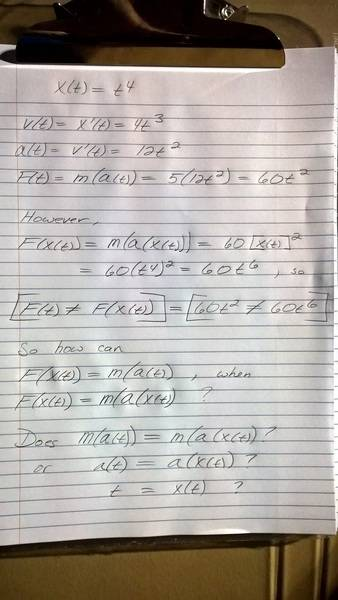Why do I arrive at 60t^2 for f(t)
and arrive at 60t^6 for f(x(t))?

It seems like f(t) = 60(t)^2
while f(t(x)) = f(t^4) = 60(t^4)^2 = 60t^6

Last edited:
Svein
You need to fix up your formula. If x is a distance and t is time you cannot have x=t4 (the dimensions do not match). You could get away with x = Ct4, but then the dimension of C would be m/s4.

As to the rest of your questions: Of course you cannot have F(t) = F(x(t)). The dimensions do not match! If you have a well-behaved function, you can possibly invert the x(t) to t = g(x), and then you will have F(t) = F(g(x)).

•Ocata
You need to fix up your formula. If x is a distance and t is time you cannot have x=t4 (the dimensions do not match).

Yes, x is a distance and t is time, so x≠t and x≠ $t^{4}$ , however, I am convinced I included parenthesis everywhere to indicate that x is a function of t. That is, x(t) = t and x(t) = $t^{4}$. As a function, the dimensions don't have to match, right? That is, displacement is a function of time, then x(t) = t can be written. I do not see where I may have written x = t or x = $t^{4}$

As to the rest of your questions: Of course you cannot have F(t) = F(x(t)). The dimensions do not match! If you have a well-behaved function, you can possibly invert the x(t) to t = g(x), and then you will have F(t) = F(g(x)).

Okay, and if I cannot have F(t) = F(x(t)), then why does the formula in the original post say: F(x(t)) = m(a(t)) = m v'(t) ? <---this is what is written in my book.

So I'm ending up at a contradiction. On one hand, F(x(t)) ≠ F(t), but then my book states F(x(t)) = m(a(t)). Can this be?

Thank you

Last edited:
Nathanael
Homework Helper
As a function, the dimensions don't have to match, right?[/itex]
When you're speaking of an equation which is physically meaningful, the dimensions must match. With that said, there is no need to worry about dimensions when contriving a particular example like this, because you can assume a particular unit system which leaves the dimensional-constant with unit value.
On one hand, F(x(t)) ≠ F(t),
You could say F(x(t))=F'(t) where F' is some other function (not the same as F unless x=t).
but then my book states F(x(t)) = m(a(t)). Can this be?
That's what I see it as saying: some function F of x of t is equal to (m times) some other function a(t).

It's really not an interesting statement (and isn't relevant to the Work-KE theorem).

It also doesn't imply a(x(t))=a(t) (which is the source of your contradiction).

P.S. (t4)2=t8 (not t6)

•Ocata
Nugatory
Mentor
In your example, you went wrong in the step where you wrote $$F(x(t)) = m(a(x(t))) = 60[x(t)^2]^2$$ The first equality is correct, the second is not. The problem is that ##a(t)## and ##a(x)## are different functions: the first is the formula you use to calculate the acceleration at a given time and the second is the formula you use to calculate the acceleration when the object is at a given position and is not equal to ##12t^2##. Whenever you see ##a(t)## you are allowed to substitute ##12t^2##, but you cannot make the same substitution when you see ##a(x)##.

The same is true of ##x##, ##v##, and ##F##; for example ##x(t)=t^4## but ##x(x)=x##.

If you work through your example again but use different names (for example ##F_x(x)## and ##F_t(t)## for the force) for the functions of time and of position it will all come out consistently.

•Ocata
DrGreg
Gold Member
Many physics authors and teachers use a convention where, for example, ##F(x)## denotes a force as a function of distance, and ##F(t)## denotes the same force as a function of time. Strictly speaking, from a pure mathematician's point of view, this is nonsense and you should really use different names for the two functions, e.g. ##F_1(x)## to denote force as a function of distance, and ##F_2(t)## to denote force as a function of time. With this notation we have$$F_2(t) = F_1(x(t))$$

Last edited:
•Ocata and PeroK
Thank you,

If I were to look at each function graphically, I have something like this: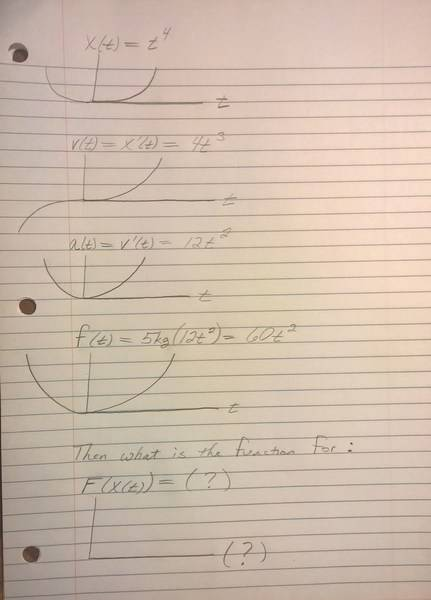However, I'm having some difficulty in figuring out how to express the force as a function of distance.

From starting with a displacement as a function of time: x(t) = $t^{4}$ and arriving at a force with respect to time: f(t) = $60t^{2}$

How can I take it one step further and find a function of force with respect to displacement? F(x) or F(x(t)) ?

My first guess is something like this: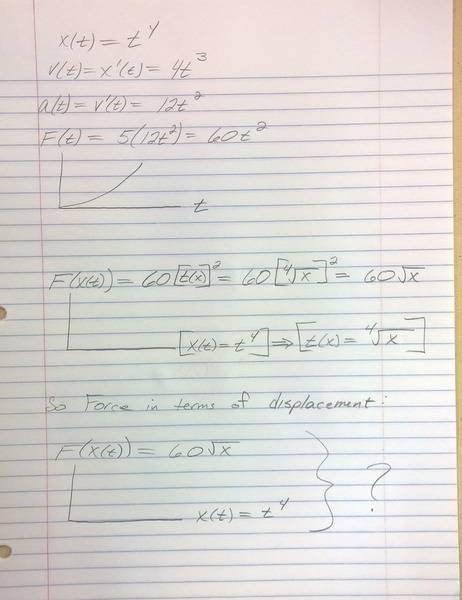Svein
Well, first we introduce a dimension-correcting factor B (with dimension m/s4), so that $x(t)=Bt^{4}$. For x≥0, this is one-to-one, so $t=(\frac{x}{B})^{-4}$.
•